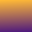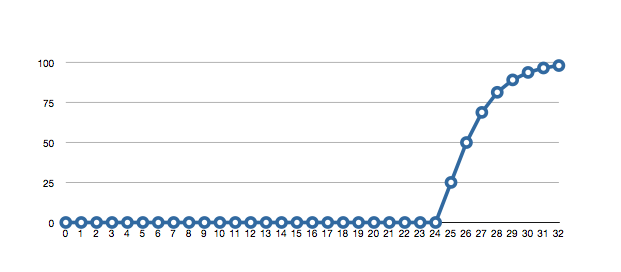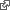#Fear of Floats

Highly optimized floating point has lead more language designers to make it the sole numerical representation. This raises the question, how large can an integer be and still be reliably stored in these systems?

Peter Denning suggests that there is always a "semantic gap" between the problems being solved in a given era and the optimization of hardware to solve them. He cites floating point as an early example of such a gap and its haphazard closing through various formats with various problems. IEEE standard have smoothed many of these but with a residual complexity that is daunting.

Programmers are cautioned to not compare floats for equality. Rounding noise is said to creep in which will throw exact comparisons off. This is often true, but not always. The mantissa has a width in bits and these bits will behave like integers so long as exponent precision and normalization practices allows.

The CDC-6000 series computers supported unnormalized floating point instructions. In this case the hardware did less so that programmers could do more, a strange twist in the semantic gap.

### Unique Identifiers

I found the need to generate random numbers to be used as unique identifiers. Since these would pass through single precision IEEE floating point representation, I wondered, how big can they be? Bigger means less chance of a collision; too big and they won't pass safely.

A quick read of the wikipedia page lead me to believe 9 decimal digits would pass through the 23 bit mantissa. This doesn't work out. 2^23 is much less than 10^9. Rather than read more carefully I chose instead to test a few IEEE implementations.

I ran a million trials each for random integers of various widths. This answered two questions at once: could the random number generate the bits and could floating point pass them reliably.

long p = (long)(rand()%w); long q = (long)(float) p;

long p = (long)(Math.random()*w); long q = (long)(float) p;

Coding in both c++ and java, I chose a random p less than w, converted it to float and back to integer q. In both languages this worked reliably for w <= 2^24.

### Error RatesSingle precision IEEE floating point error rates for random numbers of a given bit width computed in java.

Both languages produced errors, p != q, at the same rate as w increased. 25% errors at 2^25, 50% at 2^26.

There are several mysteries here. Why is 2^24 ok when the mantissa is only 23 bits wide? Also, when our random numbers are too wide by one bit, why don't half of them fail, why only one quarter?

I returned to wikipedia and read more carefully with specific questions. wikipediaThe IEEE 754 standard specifies a binary32 as having:

• Sign bit: 1 bit
• Exponent width: 8 bits
• Significand precision: 24 bits (23 explicitly stored)

This gives from 6 to 9 significant decimal digits precision (if a decimal string with at most 6 significant decimal is converted to IEEE 754 single precision and then converted back to the same number of significant decimal, then the final string should match the original; and if an IEEE 754 single precision is converted to a decimal string with at least 9 significant decimal and then converted back to single, then the final number must match the original).

• My own test showed that 7 digit number pass through fine and that 8 digit numbers don't. The 9 I had seen before was for passing through decimal representation and then back to float. Not the right number. Elsewhere I found the decimal precision quoted as 7.22 digits which agrees with experiment. wikipedia### Significands

Wikipedia chose to use the term significand where I would say mantissa. What's with that? I clicked and learned, this use of mantissa is discouraged by the IEEE floating-point standard committee because it conflicts with the pre-existing use of mantissa for the fractional part of a logarithm. wikipediaThe same article explained how I could get 24-bits of precision out of a 23-bit significand, because the most significant bit is always 1 for a normalized number, this bit is not typically stored and is called the "hidden bit".

Seymour Cray didn't give us extra mantissa bits with his unnormalized arithmetic. That IEEE complexity gives us something. wikipediaWith the leading one bit omitted, the next bit in half of my random numbers is zero. Normalization shifts making room. This explains why when I choose random numbers too wide some still fit. The error rate depends on the chance of a suitable run of zeros to let the once-big number still fit.

### Why Experiment

With something as well studied at floating point shouldn't we just know the answers? I think not. Knowledge tied to need comes more quickly and lasts much longer.

My analysis of floating point numbers also doubled as a test of the native random number generators in the two languages I tested. In my application I need simple and portable generators that are known (or can be tested) to produce sufficiently wide results.

I ran both tests well beyond 2^24 and found both sufficient but not the same. The built-in rand() in my c++ implementation ran 32 bits wide. My java generator, much wider. I can tell where bits ran out because error rates stopped growing.

I explored widths expanding in both powers of two and powers of ten. I geometrically increment w in a for loop by two or ten.

for (long w=1; w<=10000000000000L; w*=2) { ... }

for (long w=1; w<=10000000000000L; w*=10) { ... }

This loop runs over a similar range of widths even as I changed the step rate, better than counting iterations. I found working in decimal preferable until I wanted to study exactly where space for bits ran out.

TextMate knew how to run both c++ and java with a single keystroke. No run took longer that a couple of seconds. If it matters to you, c++ was half again faster than java.